# The QLIM Procedure

### Prior Distributions

Subsections:

The PRIOR statement is used to specify the prior distribution of the model parameters. You must specify a list of parameters, a tilde ~, and then a distribution with its parameters. You can specify multiple PRIOR statements to define independent priors. Parameters that are associated with a regressor variable are referred to by the name of the corresponding regressor variable.

You can specify the special keyword _REGRESSORS to consider all the regressors of a model. If multiple prior statements affect the same parameter, the prior that is specified is used. For example, in a regression with three regressors (X1, X2, X3) the following statements imply that the prior on X1 is NORMAL(MEAN=0, VAR=1), the prior on X2 is GAMMA(SHAPE=3, SCALE=4), and the prior on X3 is UNIFORM(MIN=0, MAX=1):

...
prior _Regressors ~ uniform(min=0, max=1);
prior X1 X2 ~ gamma(shape=3, scale=4);
prior X1 ~ normal(mean=0, var=1);
...


If a parameter is not associated with a PRIOR statement or if some of the prior hyperparameters are missing, then the following default choices are considered:

Table 22.2: Default values for prior distributions.

 PRIOR distribution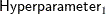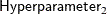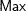Parameters Default Choice NORMAL MEAN=0 VAR=1E6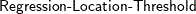IGAMMA SHAPE=2.000001 SCALE=1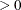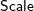GAMMA SHAPE=1 SCALE=1UNIFORMUNIFORM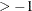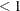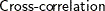BETA SHAPE1=1 SHAPE2=1T LOCATION=0 DF=3See the section Standard Distributions for density specification.

#### Priors for Heteroscedastic Models

The choice of the prior distribution for a heteroscedastic model is particularly interesting. Based on the notation provided in section HETERO Statement, you need to provide a prior for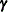. This prior is enough to induce different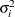into the analysis. The resulting inference is a compromise between two cases: the inference based on the entire sample and the inference based on a single unit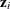. The degree of compromise is determined by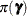.

This type of modeling is similar to a method called “hierarchical Bayes,” in which the prior is characterized by two levels: one for each individual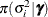and one for the entire population. In this scenario the degree of compromise between the information provided by a unit and the information provided by the entire sample is determined by the data.

The choice of the prior might not be straightforward, and it can heavily affect sampling performance. Depending on how the heteroscedastic effects are modeled, the default priors are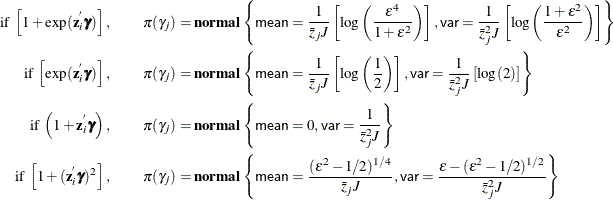where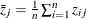,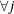, andis a small number (by default,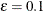for the EXPONENTIAL link function and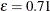for the QUADRATIC link function).

The priors for the EXPONENTIAL and QUADRATIC link functions are not straightforward. To understand the choices, do the following:

1. Assume that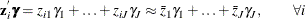2. Set the priors according to the link function type:

• For the EXPONENTIAL link function, set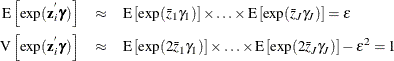Assume a normal prior for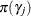, and set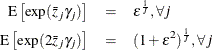Based on the properties of the lognormal distribution, the prior hyperparameters for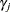can be derived. Notice that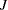is the number of regressors that are used in the heterogeneous regression. If the intercept is excluded, then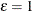.

• For the QUADRATIC link function, set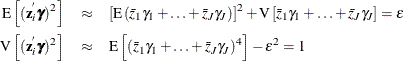Assume a normal prior for. Based on the properties of the normal distribution, the preceding expressions return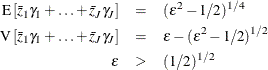The prior hyperparameters forcan be derived by setting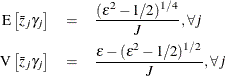Notice thatis the number of regressors that are used in the heterogeneous regression. It is important to emphasize that the restriction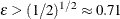is likely to introduce some distortion becausecannot be any “small” number.